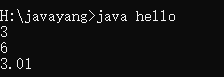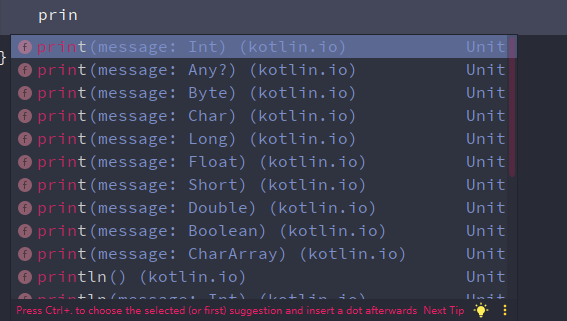# Java -- 最简单的认识重载

#### 定义

##### 示例

public class hello{
public static void main(String args[]){
System.out.println(sum(1,2));
System.out.println(sum(1,2,3));
System.out.println(sum(1.01,2.0));
}

public static int sum(int a, int b){
return a+b;
}

// 参数数量不同
public static int sum(int a, int b, int c){
return a+b+c;
}

// 类型不同
public static double sum(double a, double b){
return a+b;
}
}#### 最常见的方法重载posted @ 2019-10-29 20:51  Gyyyang  阅读(101)  评论(0编辑  收藏